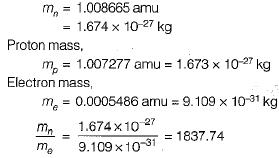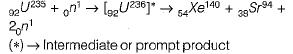Courses

# Test: Nuclear Power Plants - 1

## 10 Questions MCQ Test Topicwise Question Bank for Mechanical Engineering | Test: Nuclear Power Plants - 1

Description
This mock test of Test: Nuclear Power Plants - 1 for Mechanical Engineering helps you for every Mechanical Engineering entrance exam. This contains 10 Multiple Choice Questions for Mechanical Engineering Test: Nuclear Power Plants - 1 (mcq) to study with solutions a complete question bank. The solved questions answers in this Test: Nuclear Power Plants - 1 quiz give you a good mix of easy questions and tough questions. Mechanical Engineering students definitely take this Test: Nuclear Power Plants - 1 exercise for a better result in the exam. You can find other Test: Nuclear Power Plants - 1 extra questions, long questions & short questions for Mechanical Engineering on EduRev as well by searching above.
QUESTION: 1

Solution:
QUESTION: 2

Solution:
QUESTION: 3

### Atomic number of an element in the periodic table represents the numbers of

Solution:
QUESTION: 4

The mass number of a substance represents the sum of total number of

Solution:
QUESTION: 5

Which of the following is not identical for an atom and an isotope?

Solution:
QUESTION: 6

The mass of electron as compared to that of neutron is

Solution:

Neutron mass,QUESTION: 7

Which of the following energy acts as a glue which binds the protons and neutrons together in the nucleus?

Solution:

The energy associated with the mass defect is known as the binding energy of the nucleus. This energy acts as a ‘glue’ which binds the protons and neutrons together in the nucleus.

QUESTION: 8

How many fast neutrons are produced on the average as a product of reaction of each fission of U-235?

Solution:QUESTION: 9

The energy released during the fission of one atom of U-235 in MeV is about

Solution:

When any uranium atom undergoes a fission process, about 2.5 neutrons having kinetic energy of the order of 2 MeV are generally produced and the energy generated by fission is of the order of 200 MeV per atom fissioned.

QUESTION: 10

Uranium 238 is represented as 92U238. What does it mean?

Solution:

ZXA means
A = mass
= number of proton + number of neutron
Z = atomic number = number of protons
∴ Z = 92 = np
and nn= 238 - 92 = 146